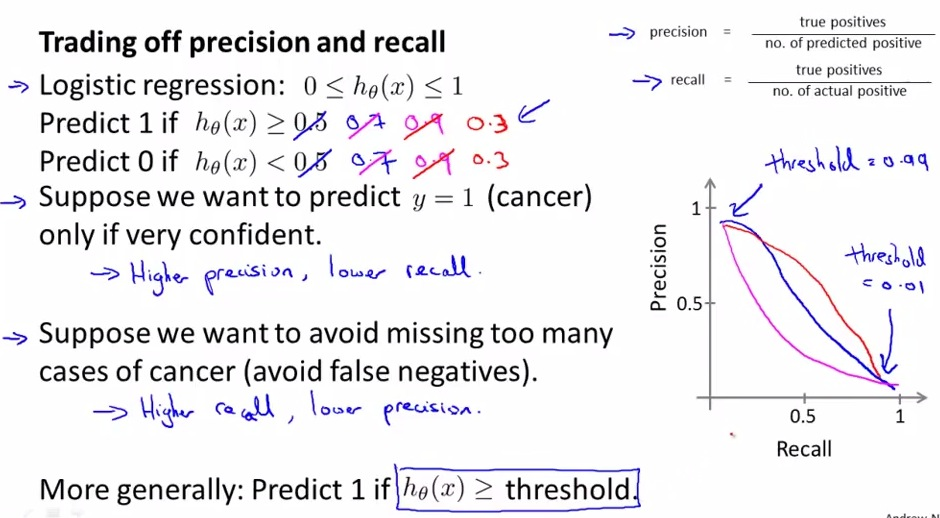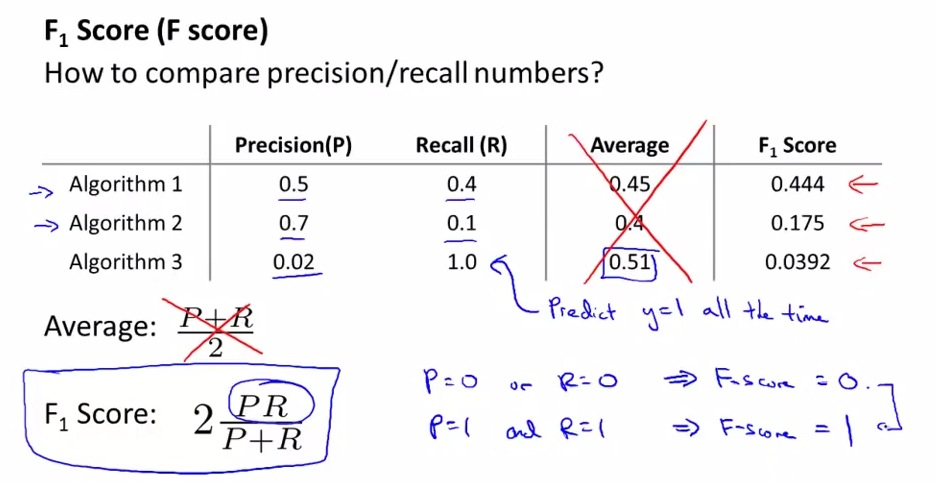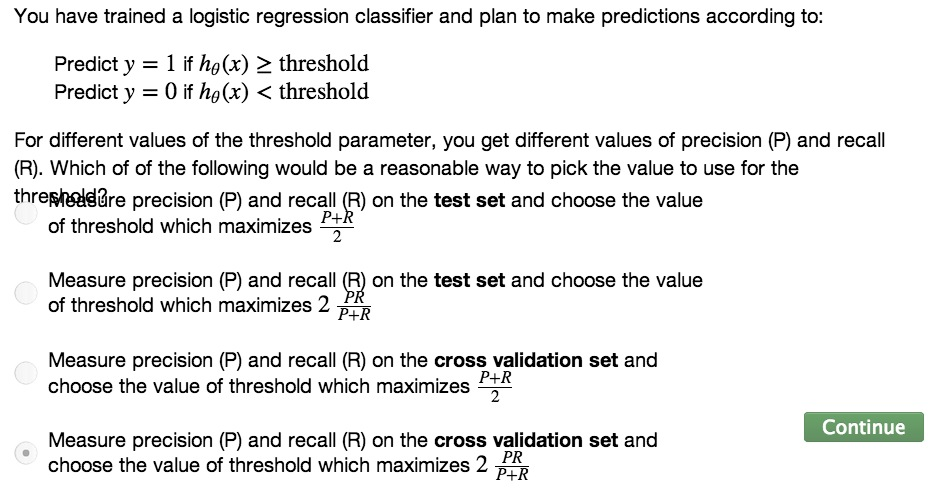• Previous: Precision & Recall as evaluation metrices to analyze learning algorithm with skew classes (data)
• balance the control of precision & recall, what are the trade-off. and how to effectively use the value for the learning algorithm• For cancer classifier problem there two possible cases for precision/recall trade-off.
• Either tell them that they have a cancer(would giving a shock to them if we tell them they have a cancer and must run painful treatment). Must be really confident(Higher precision, lower recall),
• Or we don't want to tell them they don't have cancer while they actually have(lower precision, higher recall).
• To tell someone a cancer, it has to really confident
• To increase confidentiality, increase the precision to >= 0.7, then the recall will be lower.
• Even so, it also dangerous to tell someone doesn't have a cancer, when they actually do.
• The precision then will be decrease to >= 0.3, but the recall will be higher
• More precision == higher threshold, More recall == lower threshold
• Choosing threshold automatically?• Remember single row number evaluation metrics? use a number to cv to observe how the algorithm doing
• The number that mentioned could make decision making for algorithm a lot easier. But that is not the case when we have two number indication(precision and recall), instead of just one.
• Compute average of both number is one method,but not a recommended solution.
• In this case, the average will be highest in algorithm no.3, where we set y=0/y=1 all the time, which is not a good classifier.
• use F1 Score, a better way than just computing average (calculating the weight for both P/R).
• The name only convention, not have particular meaning
• The intermediate value between 0 and 1 still give a reasonable value.• Use cross validation set because we want to pick the best parameters(threshold) that have the highest F1 score.
• This video discuss about which is to weight more P/R
• Also F1 Score used to set the right threshold.
• Try various range of threshold, use it to cross validation set, then analyze which has a better value with F1 score.